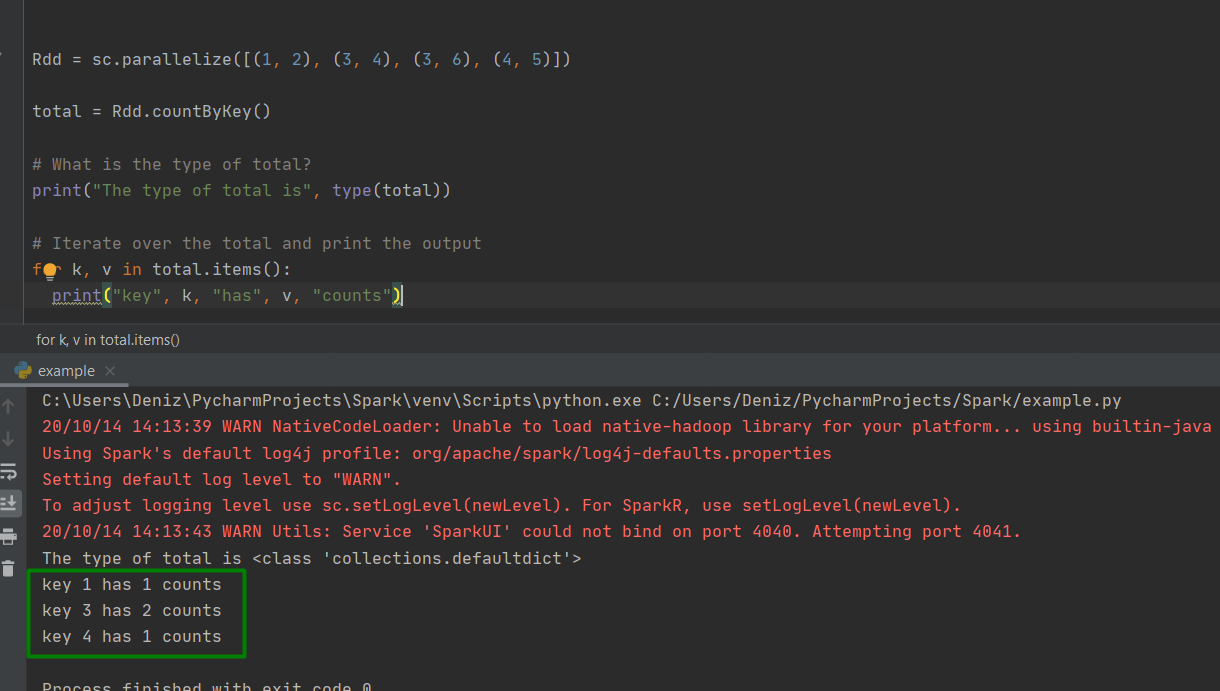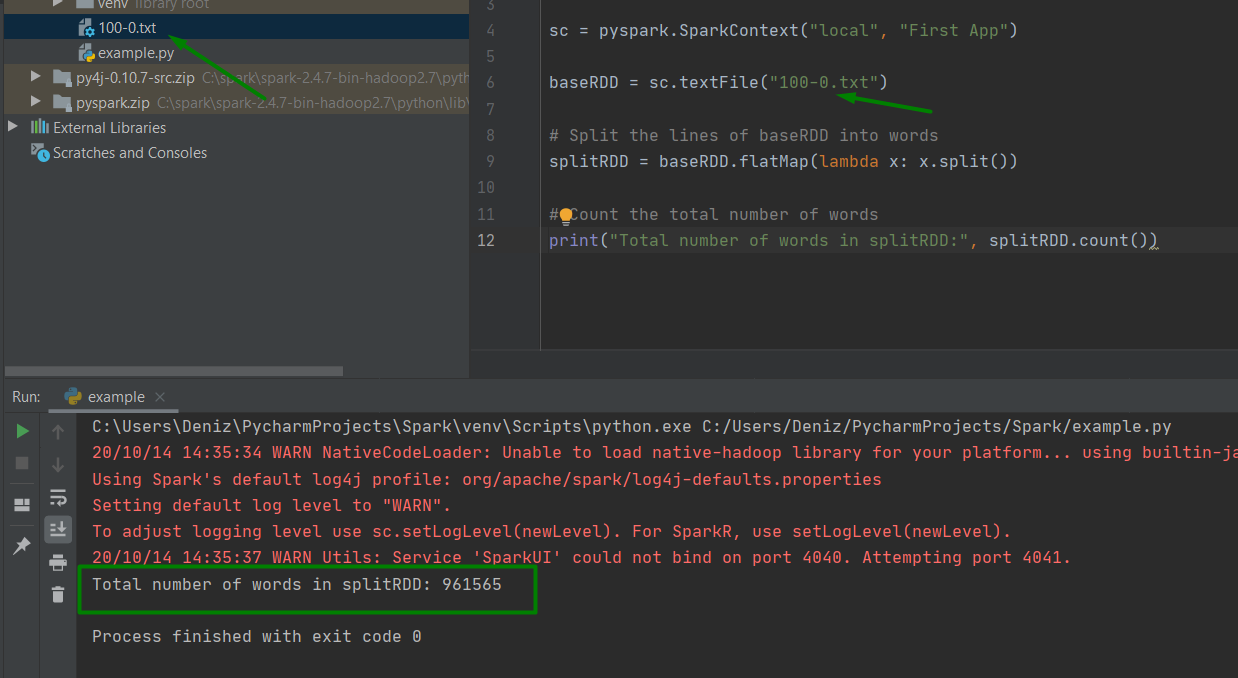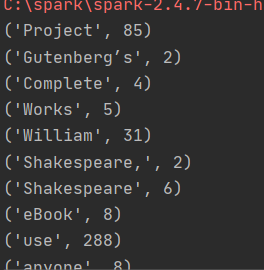### reduce() action

• reduce(func) action is used for aggregating the elements of a regular RDD.
• The fucntion should be commutative (changing the order of the operands does not change the result) and associative.

### saveAsTextFile() action

• saveAsTextFile() action saves RDD into a text file inside a directory with each partition as a separate file.

### countByKey() action

• countByKey() only available for type(K,V)
• countByKey() action counts the number of elements for each key

### collectAsMap() action

• collectAsMap() return the key-value pairs in the RDD a dictionary

## Example

### CountingBykeys

```Rdd = sc.parallelize([(1, 2), (3, 4), (3, 6), (4, 5)])

total = Rdd.countByKey()

# What is the type of total?
print("The type of total is", type(total))

# Iterate over the total and print the output
for k, v in total.items():
print("key", k, "has", v, "counts")

```### Create a base RDD and transform

Here are the brief steps for writing the word counting program:

• Create a base RDD Complete_Shakespeare file.
• Use RDD transformation to create a long list of words from each element of the base RDD.
• Remove stop words from your data.
• Create pair RDD where each element is a pair tuple of (“w”,1)
• Group the elements of the pair RDD by key (word) and add up their values.
• Swap the keys (word) and values (counts) so that keys is count and value is the word.
• Finally, sort the RDD by descending order and print the 10 most frequent words and their frequencies.

```baseRDD = sc.textFile("100-0.txt")

# Split the lines of baseRDD into words
splitRDD = baseRDD.flatMap(lambda x: x.split())

# Count the total number of words
print("Total number of words in splitRDD:", splitRDD.count())
``````for word in resultRDD.take(10):
print(word)

# Swap the keys and values
resultRDD_swap = resultRDD.map(lambda x: (x, x))

# Sort the keys in descending order
resultRDD_swap_sort = resultRDD_swap.sortByKey(ascending=False)

# Show the top 10 most frequent words and their frequencies
for word in resultRDD_swap_sort.take(10):
print("{} has {} counts". format(word, word))

```See you in the next article..

7,010 views last month,  1 views today